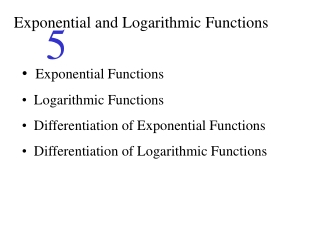DownloadDownload PresentationExponential and Logarithmic Functions

# Exponential and Logarithmic Functions

Télécharger la présentation## Exponential and Logarithmic Functions

- - - - - - - - - - - - - - - - - - - - - - - - - - - E N D - - - - - - - - - - - - - - - - - - - - - - - - - - -
##### Presentation Transcript

1. Exponential and Logarithmic Functions 5 • Exponential Functions • Logarithmic Functions • Differentiation of Exponential Functions • Differentiation of Logarithmic Functions

2. (5.1): Exponential Function An exponential function with base b and exponent x is defined by y Ex. x y Domain: All reals Range: y > 0 (0,1) 0 1 x 1 3 2 9

3. Laws of Exponents Law Example

4. Properties of the Exponential Function • The domain is . • 2. The range is (0, ). • 3. It passes through (0, 1). • 4. It is continuous everywhere. • 5. If b > 1 it is increasing on . If b < 1 it is decreasing on .

5. Examples Ex. Simplify the expression Ex. Solve the equation

6. (5.2): Logarithms The logarithm of x to the base b is defined by Ex.

7. Examples Ex.Solve each equation a. b.

8. Laws of Logarithms Notation: Common Logarithm Natural Logarithm

9. Example Use the laws of logarithms to simplify the expression:

10. Logarithmic Function The logarithmic function of x to the base b is defined by Properties: 1. Domain: (0, ) • Range: 3. x-intercept: (1, 0) 4. Continuous on (0, ) 5. Increasing on (0, ) if b > 1 Decreasing on (0, ) if b < 1

11. Graphs of Logarithmic Functions Ex. y y (0, 1) (0, 1) x x (1,0) (1,0)

12. Ex. Solve Apply ln to both sides.

13. (5.4): Differentiation of Exponential Functions Derivative of Exponential Function Chain Rule for Exponential Functions If f (x) is a differentiable function, then

14. Examples Find the derivative of Find the relative extrema of + – + x Relative Min. f (0) = 0 -1 0 Relative Max. f (-1) =

15. (5.5): Differentiation of Logarithmic Functions Derivative of Exponential Function Chain Rule for Exponential Functions If f (x) is a differentiable function, then

16. Examples Find the derivative of Find an equation of the tangent line to the graph of Slope: Equation:

17. Logarithmic Differentiation • Take the Natural Logarithm on both sides of the equation and use the properties of logarithms to write as a sum of simpler terms. • Differentiate both sides of the equation with respect to x. • Solve the resulting equation for .

18. Examples Use logarithmic differentiation to find the derivative of Apply ln Properties of ln Differentiate Solve